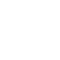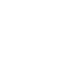The latest research & treatment news about Hepatitis C infection, diagnosis, symptoms and treatment.by: Albrecht Ernst

Your viral load is the amount of specific viruses that you have, in a given volume of your blood (usually 1 milliliter = 1 cubic centimeter). More precisely, it means that the amount of Hep C genetic material found in your blood corresponds to as many Hep C viruses as the given number says. Therefore the given number denotes “viral equivalents.”

There appears to be no significant correlation between HCV RNA levels and ALT values or histological activity in patients untreated by anti-viral therapies (Interferon). Viral load varies between infected individuals but is not a useful prognostic indicator nor does it measure the severity of virus-induced liver disease.

WHAT DOES NEGATIVE OR “NOT DETECTED” MEAN?

The viral load can range from “not detected” to hundreds of millions. The meaning of “not detected” or “negative” differs, depending on the test used. In one lab, the detection limit for the *quantitative* HCV RNA test by *PCR* is 200 virus equivalents/ml (and with the *qualitative* test they can detect down to 10 virus equivalents/ml). The less expensive quantitative *bDNA* test has a detection limit of about 200,000 virus equivalents/ml. So it is less sensitive, but above its detection limit it is more accurate than the PCR test.

So, when you are “negative”, maybe you have no Hepatitis C virus in your blood. But maybe also, you do have Hepatitis C virus in your blood, but the number of viruses is lower than the detection limit. {Example: If the less expensive quantitative *bDNA* has been used, and the detection limit is 200,000 virus equivalents/ml, any number less than this would register as “negative” or “not detected”, when in fact, the viral load could be present, but less than this detection limit.} Your lab can tell you which testing measure is used, and your doctor can explain what it means in your case.

WHAT DOES “POSITIVE” MEAN?

When you get back the result of your HCV RNA quantitative test, and when the lab was able to determine the amount of virus in your blood, then it is important to write down not only the number, but also in what units this number is given.

I) Volume

The volume of blood, that the number refers to, is usually one milliliter.

But some labs give the number for 20 microliters = 1/50 milliliter. So in these cases you have to multiply the result of the viral load by 50 to get the number for 1 milliliter.

II) Amount of Virus

Unfortunately, there are several ways to express the viral load. So, in order to be able to compare different results, you have to know how to convert these numbers to some standard format, which let’s say is just the plain number of viruses per milliliter, like 1.5 Million/ml, or 1,500,000/ml. (both of these numbers are the same).

a) Measure by weight

Sometimes, the lab reports the amount of genetic material found by its weight. 1 pg (pico-gram) of genetic material corresponds to about 1 million virus equivalents, so, if your lab result is given in picograms, just multiply the lab result by 1,000,000, and you have the number of viruses.

b) Measure by virus count

i) Plain numbers

Often the virus count is expressed as a plain number, like 1.73 million, or 1,730,000 or 1730000. Millions sometime are abbreviated by the prefix “M” (Mega). So when you see 1.73 Meq/ml, it means 1.73 Mega-equivalents/ml or again 1730000 equivalents/ml..

ii) Exponential format

Large numbers are often expressed in exponential form, that means a number, multiplied by 10 with an exponent. To convert this to normal numbers, append as many zeroes to a “1” as the exponent says, and multiply this with the number. In some lab report, the viral load was “Hep C RNA Quant 17.3 x 10(exp) 5 equivalents/ml”. So, with 5 as exponent, you have to append 5 zeroes to a “1”, that gives 100000, and multiply this with the number 17.3, that gives 1730000 as the viral load. Normally this would be written 1.73×10(exp)6, or 1.73×106 , (which are again the same number).

17.3×105 = 1.73×106 = 1,730,000

iii) Logarthmic format

Now, recently some people express these numbers also in logarithmic form (logarithmic transformed number).

log(1730000)=6.24

6.24 is the logarithmic transformed number of the viral load of our above example. A result of 3.5 for a viral load, that someone reported, seems to be such a number (unless he forgot to write down a “10” and an exponent). You need a calculator to convert this. You have to use the function 10x , where you have to replace x with the logarithmic number, in the above case 3.5. The result would be: 103.5 = 3162 virus equivalents per milliliter.

When you take the logarithmic number from the first example, 6.24, you have to calculate 106.24 = 1730000 , and here we have the original number of virus equivalents again. If you don’t have a calculator, you can estimate the order of magnitude of a viral load expressed as a logarithmic number. From the logarithmic number, you take the first digit (left of the point) and add 1 to this number. This gives you the number of digits that your viral load has (expressed as a plain number).

Example: Logarithmic number 6.24

Left of the point is “6”. 6+1 = 7

The number that gives the viral load is 7 digits long, that means it is between 1,000,000 and 9,999,999 (digit # 1 234 567)

The next digit (right of the point of the logarithmic number) shows whether you are high or low in the range.

In case you have a logarithmic number *and* a blood volume other than 1 ml, you have to convert the logarithmic number to a plain number *first*, and then correct it to correspond to 1 ml !

Therefore it is important to have a close look at your lab report and see in what units the result is given!

There is still no general agreement on what Viral Load is considered low and what is high in Hepatitis C. This interpretation makes sense for people not currently being treated – for someone who is 6 months into an INF + RIBA trial, even 200,000 could be considered a high titer.

(Numbers are Virus Equivalents per Milliliter)

below 200,000 very low (undetectable by *bDNA* test)

200,000 to 1,000,000 low

1,000,000 to 5,000,000 medium

5,000,000 to 25,000,000 high

above 25,000,000 very high

Once again, please note that this information is not written by an MD or medical expert. Nothing can (or should) take the place of appropriate medical care.